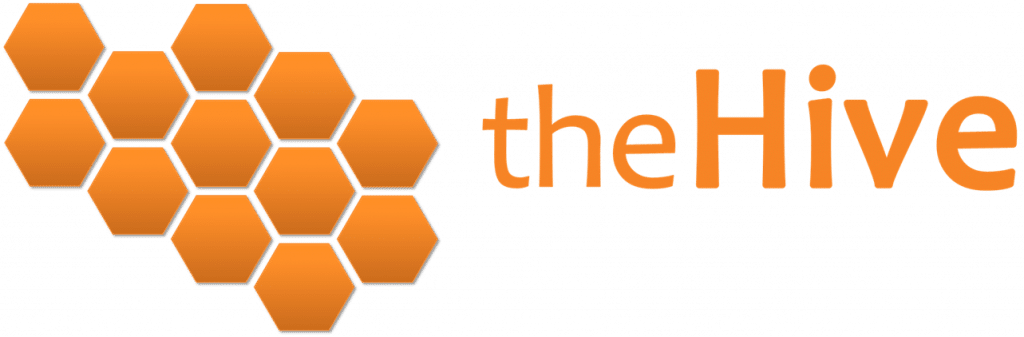## Errors of Predictive Models

You’ve created a bunch of predictive models and you need to measure the accuracies of each of them to choose the one that’s best for your purposes.

In this course I’m going to show you all of the most used measures and teach you exactly when to use them – and when not to.

Most importantly I’m going to show you how to interpret each of them, so you always know which is the best measure for your uses.

Video lessons

Practice sessions

Certificate of completion

Interactive experience

Perfect for beginners

• Description
• Content
• LEARNING Outcomes
• FORUM
• CertificatE

So you’ve created a bunch of predictive models and now you need to choose the one that’s best for your purposes.

How do you do this? Eeny meeny? Throw darts at them?

Probably not.

You need to be able to measure the accuracies of each of your models so you can choose between them objectively.

The only problem is that there are literally loads of different measures you can use. Reading around the internet and watching videos on YouTube will only make you more confused, because each method has its own set of dedicated fans.

In this course I’m going to show you all of the most used measures and show you exactly when to use them and – more importantly – when not to.

And there’s only one place in the universe that you can get this course – right here!

If you’re truly interested in measuring the effectiveness of your models, don’t miss this course!

#### Here's what you'll learn:

1.

you’ll learn why most papers textbooks and articles get the terminology wrong – and how to get it right

2.

you’ll learn how to calculate the errors of regression-based predictive models – with lots of practice sessions

3.

you’ll learn how to calculate the errors of classification-based predictive models –lots more practice sessions

4.

you’ll learn exactly which statistical tests to use, why, when and how – and how to interpret them correctly too!

1

#### Introduction to the Errors of Predictive Models

An introduction to this course and what to expect as you learn from the video lessons and practice exercises

Open Access

##### CHAPTER HIGHLIGHTS

Introduction to the course

2

#### Errors of Regression Models (Part 1)

In this chapter you'll learn the building blocks of calculating the variability of your data using R-Squared and Variance

Open Access

Free Plan

##### CHAPTER HIGHLIGHTS

Introduction to the Errors of Regression-Based Predictive Models

Errors, Residuals, Deviations

Residuals

R-Squared

Variance

3

#### Errors of Regression Models (Part 2)

In this chapter you'll learn the building blocks of calculating the bias of your data, why how and when to use each measure

Free Plan

##### CHAPTER HIGHLIGHTS

Mean Absolute Error

Mean Error

RMSE

Recommendations

4

#### Errors of Classification Models (Part 1)

In this chapter you'll learn how to measure the accuracy of your models - and why you need additional measures!

##### CHAPTER HIGHLIGHTS

Introduction to the Errors of Classification-Based Predictive Models

Confusion Matrix

PPV & NPV

Sensitivity & Specificity

Accuracy

5

#### Errors of Classification Models (Part 2)

In this chapter you'll learn about the additional measures you need, how to calculate them, when and why!

##### CHAPTER HIGHLIGHTS

F-measure & Youden’s J

ROC Curves

AUC & Gini Coefficient

Multi-Class Classifications

Recommendations

6

#### Course Recap

In this chapter you'll recap everything you've learnt about assessing regression-based and classification-based predictive models